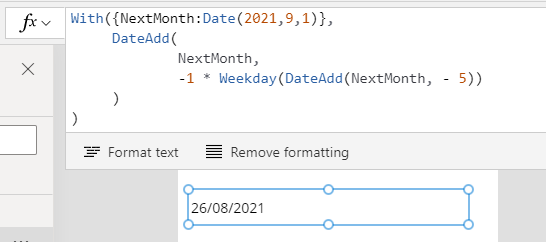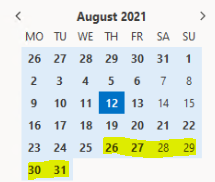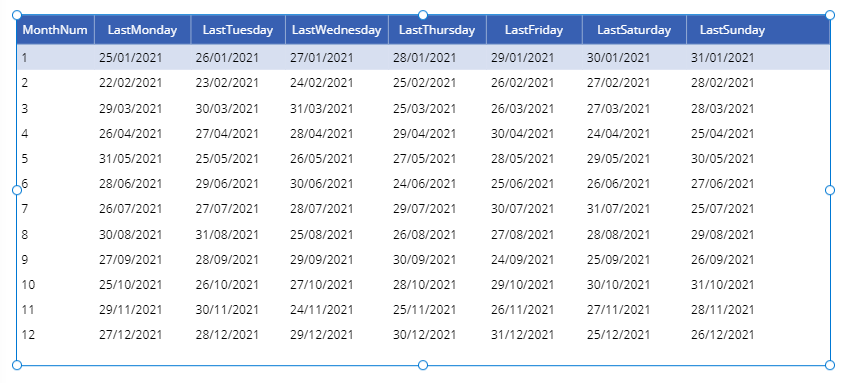# Dates - How to get the last weekday for a month

When building apps, it can be very useful to determine the last workday in a month. For example, what's the last Thursday in a month? This post describes the formula to carry out this task.

It can be very useful to determine the last workday in a month. A typical use case scenario is to help build scheduling apps, where we may want to create reoccurring events for a given day in a month - for example, the last Monday/Tuesday/Wednesday/Thursday/Friday/Saturday or Sunday of a month.

## Generic Formula - get the last Thursday for a given month

The formula to return the last Thursday for August 2021 looks like this:
`With({NextMonth:Date(2021,9,1)},     DateAdd(             NextMonth,             -1 * Weekday(DateAdd(NextMonth, - 5))     ))`
Note that with this formula, 5 refers to Thursday  (weekday 1 is Sunday).

The screenshot beneath verifies the result - the last Thursday on August 21 is 26th August 2021.## Explanation of technique

The method we use is to start with the first day of the next month. We take the target weekday and calculate the number of days that we need to roll back.

Taking the example of the last Thursday of August 2021, we would need to roll back 6 days from September 1st to arrive at our target date.We calculate the number of days to roll back by subtracting the target weekday from the first day of the next month, and we then call the Weekday function on this result.

Here's the part of the formula that returns the value 6.
`Weekday(DateAdd(Date(2021,9,1), - 5))`

To derive the final result, we then subtract this value (6) from the first day of the next month (1st September 2021) to arrive at 26th August 2021.

## How to get the last weekdays for the current month

Here's a summary of how to retrieve the date of the last Monday/Tuesday/Wednesday/Thursday/Friday/Saturday or Sunday for the current month:

### 1 - Get the last Monday for the current month:

`With({NextMonth:         DateAdd(                 Date(Year(Now()),Month(Now()),1),1,Months         )      },      DateAdd(             NextMonth, -1 * Weekday(DateAdd(NextMonth, - 2))      ))`

### 2 - Get the last Tuesday for the current month:

`With({NextMonth:         DateAdd(                 Date(Year(Now()),Month(Now()),1),1,Months         )      },      DateAdd(             NextMonth, -1 * Weekday(DateAdd(NextMonth, - 3))      ))`

### 3 - Get the last Wednesday for the current month:

`With({NextMonth:         DateAdd(                 Date(Year(Now()),Month(Now()),1),1,Months         )      },      DateAdd(             NextMonth, -1 * Weekday(DateAdd(NextMonth, - 4))      ))`

### 4 - Get the last Thursday for the current month:

`With({NextMonth:         DateAdd(                 Date(Year(Now()),Month(Now()),1),1,Months         )      },      DateAdd(             NextMonth, -1 * Weekday(DateAdd(NextMonth, - 5))      ))`

### 5 - Get the last Friday for the current month:

`With({NextMonth:         DateAdd(                 Date(Year(Now()),Month(Now()),1),1,Months         )      },      DateAdd(             NextMonth, -1 * Weekday(DateAdd(NextMonth, - 6))      ))`

### 6 - Get the last Saturday for the current month:

`With({NextMonth:         DateAdd(                 Date(Year(Now()),Month(Now()),1),1,Months         )      },      DateAdd(             NextMonth, -1 * Weekday(DateAdd(NextMonth, - 7))      ))`

### 7 - Get the last Sunday for the current month:

`With({NextMonth:         DateAdd(                 Date(Year(Now()),Month(Now()),1),1,Months         )      },      DateAdd(             NextMonth, -1 * Weekday(DateAdd(NextMonth, - 1))      ))`

## Get all the last weekdays for a given year

We can extend this technique further to produce a table of all the final weekdays for all months in a year (2021 in the example beneath).

`ForAll(    Sequence(12),	With(            {                NextMonth: DateAdd(                    Date(2021,Value,1),1,Months)            },           {               MonthNum: Value,               LastMonday:                       DateAdd(NextMonth,                              -1 * Weekday(DateAdd(NextMonth,- 2))                      ),               LastTuesday:                       DateAdd(NextMonth,                              -1 * Weekday(DateAdd(NextMonth,- 3))                      ),               LastWednesday:                       DateAdd(NextMonth,                              -1 * Weekday(DateAdd(NextMonth,- 4))                      ),               LastThursday:                       DateAdd(NextMonth,                              -1 * Weekday(DateAdd(NextMonth,- 5))                      ),               LastFriday:                       DateAdd(NextMonth,                              -1 * Weekday(DateAdd(NextMonth,- 6))                      ),               LastSaturday:                       DateAdd(NextMonth,                              -1 * Weekday(DateAdd(NextMonth,- 7))                      ),               LastSunday:                       DateAdd(NextMonth,                              -1 * Weekday(DateAdd(NextMonth,- 1))                      )           }    ))`

This formula calls the Sequence function to generate a table of values from 1-12. We call the ForAll function to process each value in the sequence. For each row, we calculate the first day of the next month, and we use the formula from above to derive each final weekday for the month.

The screenshot beneath shows the output when we apply this formula to the Items property of a data table control.## Conclusion

A common requirement Is to get the last weekday for a given month. This post summarised formulas to carry out this task.